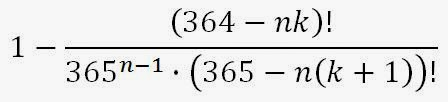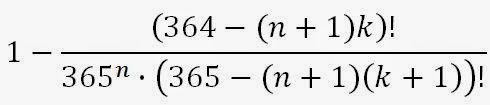## Friday, 2 May 2014

### Another Variation of the Birthday Problem

In my first post on the Birthday Problem we determined the probability of there being a shared birthday in a group of n random people. In my second post, we expanded that to look at a more general problem of having a birthday within k days time of another birthday in a group of n random people.

This time, we'll be looking at the problem of determining which person in a list is most likely to share a birthday with the people before him. In other words, if randomly selected people enter a room one at a time, which person is most likely to be the first one to share a birthday with someone already in the room? Perhaps you'd like to know because you're looking for a gambling opportunity, betting your friends on which person arriving at a party will share a birthday with someone already there.

The probability of there being a shared birthday among a randomly selected group of n people can be calculated from the expression below:Equation 1

Suppose now we add one more person. The probability of there being a shared birthday in a group of n+1 people is:Equation 2

The incremental change in the probability of there being a shared birthday in a group of n people versus n+1 people is the probability that the (n+1)th person is the first person to share a birthday with someone already in the group. So subtracting the first expression from the second gives us the probability that the (n+1)th person added to a group of n people will share a birthday with someone already.Equation 3

Now if we want to know what value of n maximizes this probability, we can plot the function to find out. I've done that here:

The peak is at n = 19. So, at 3.23%, the 20th person to enter a random group of people has the greatest chance of being the first one to share a birthday with someone already in the group.

Suppose now we repeat this analysis for the more general case of birthdays within k days time of each other. The probability of there being birthdays with k days of each other among a group of n people is:Equation 4

And in a group of n+1 people, the probability is:Equation 5

Just as before, we can subtract Equation 4 from Equation 5 to get the probability that the (n+1)th person is the first person with a birthday close to one already in the group:Equation 6

Plotting this beastly looking function will allow us to see which person entering the room is most likely to be the one having a birthday close to a birthday of a person already at the party.

The table below summarizes the information from all the peaks in the above figure.

There you have it. As random people enter a room, the 3rd is most likely to be the first to have a birthday within a month of a person already at the party. The 6th is most likely to be the first to have a birthday within a week. The 12th person is most likely to have a birthday within one day, and the 20th is most likely to have the same birthday as someone already at the party.